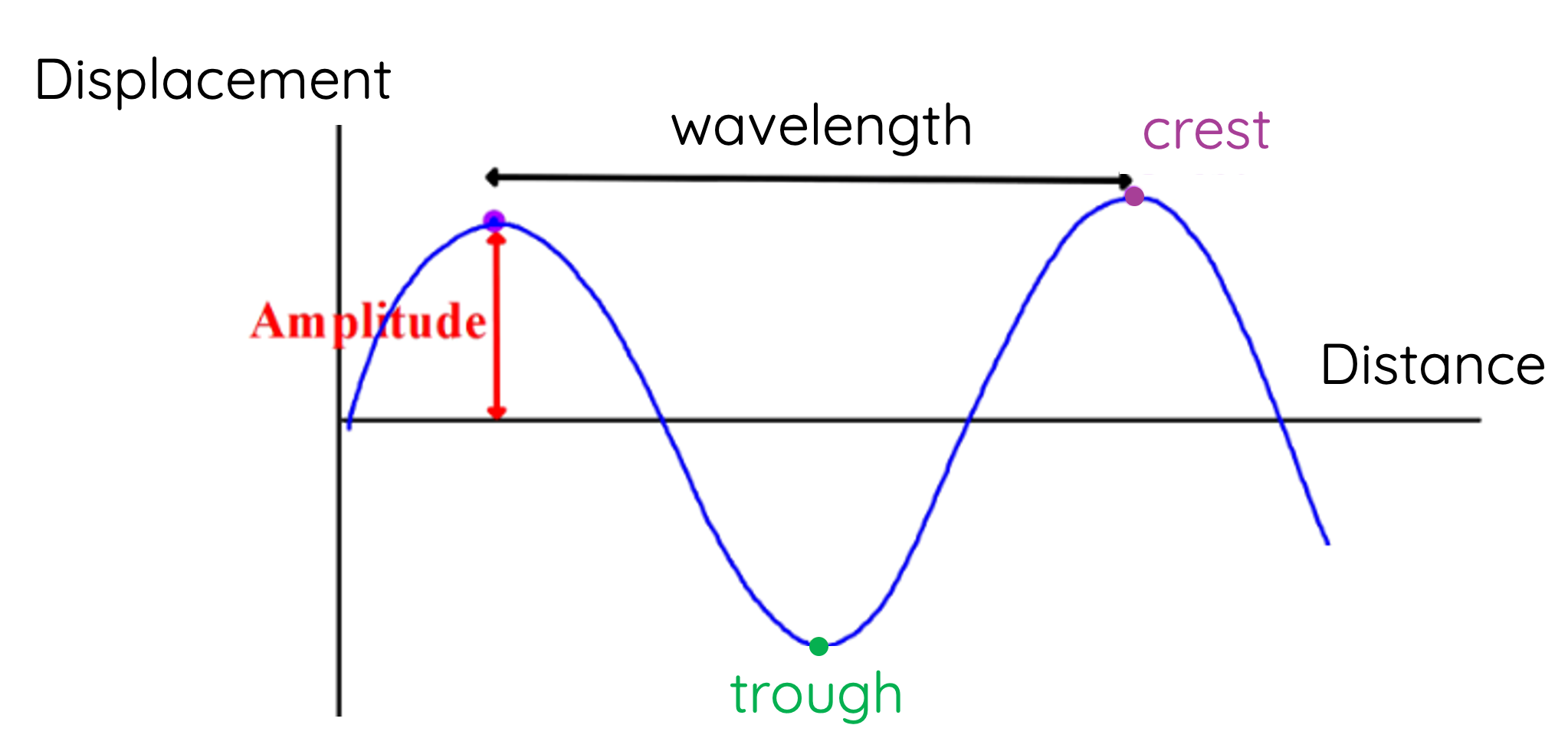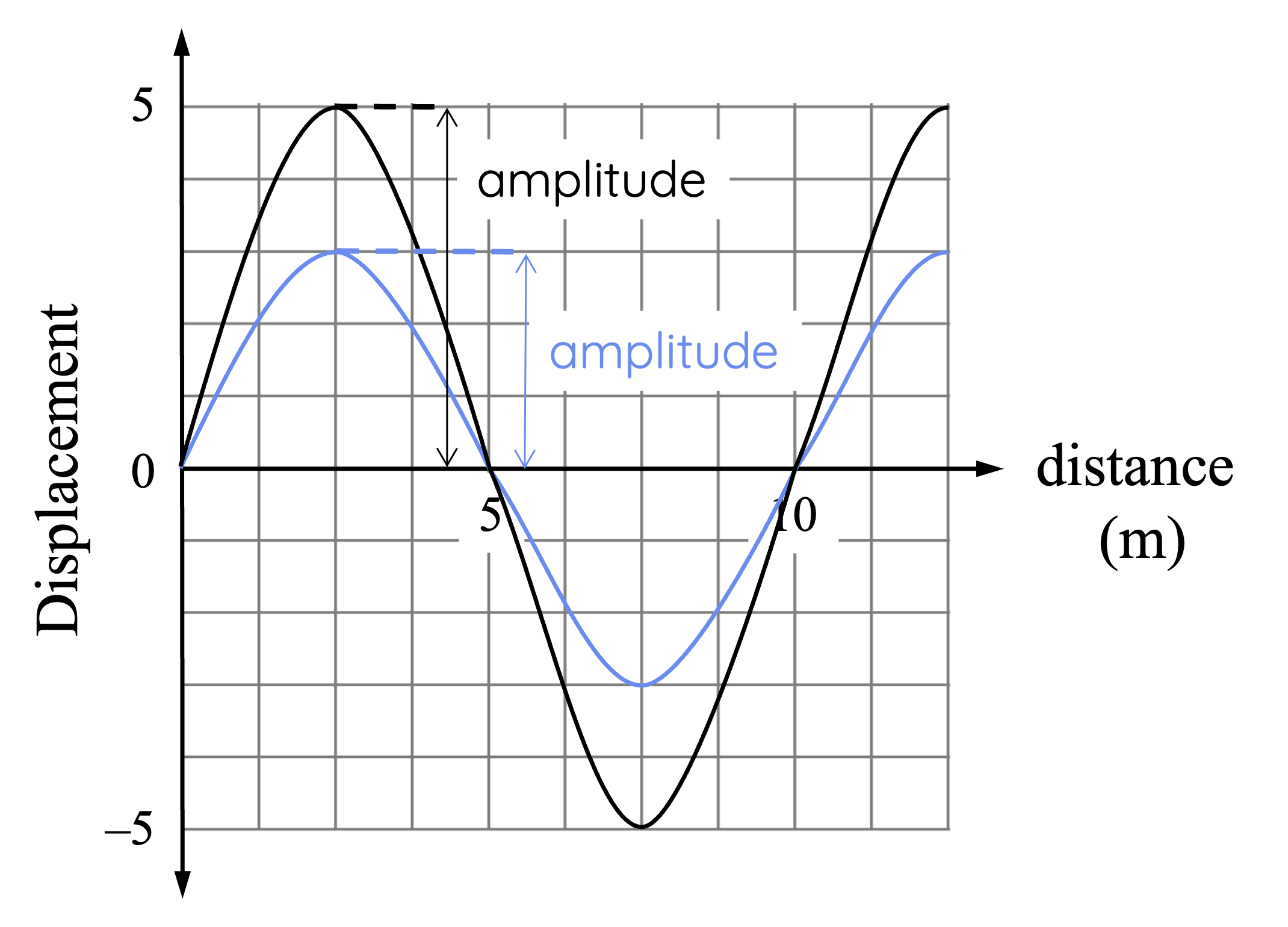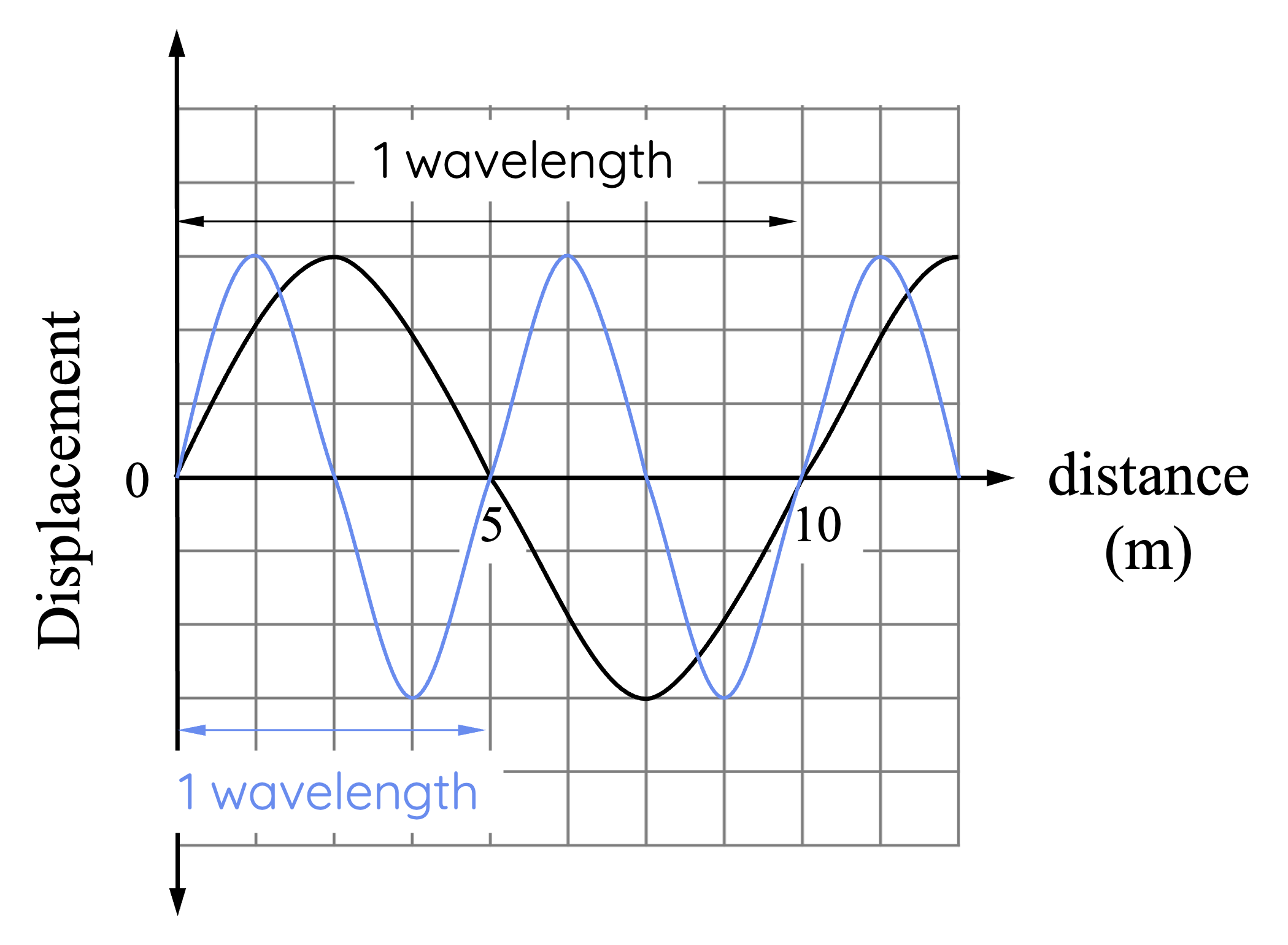# Properties of Transverse Waves

This topic is part of the HSC Physics course under the section Properties of Waves.

### HSC Physics Syllabus

• construct and/or interpret graphs of displacement as a function of time and as a function of position of transverse and longitudinal waves, and relate the features of those graphs to the following wave characteristics:

– velocity
– frequency
– period
– wavelength
– displacement and amplitude (ACSPH069)
• solve problems and/or make predictions by modelling and applying the following relationships to a variety of situations:

– v = f\lambda
– f = 1/T

### An Overview of Waves in HSC Physics

In the HSC Physics syllabus, understanding waves is fundamental, and their properties form the bedrock for many complex principles. In this article, we'll delve into the key concepts of waves including frequency, period, wavelength, amplitude, and velocity—tailored specifically for HSC physics students.

This article discusses the properties of transverse waves only. Properties of longitudinal waves are discussed separately here.

### What is a Transverse Wave?

A transverse wave is one where its direction of oscillation is perpendicular to the direction of its propagation. Examples of transverse wave include ripples on the surface of water and electromagnetic waves (e.g., light, radio waves).

### Amplitude

The amplitude is the measure of the wave's maximum displacement from its equilibrium or rest position.

A high amplitude wave carries more energy than a low amplitude wave. This fundamental concept is vital when studying electromagnetic waves, where its amplitude correlates to the brightness of light.In transverse waves, the crest, is the highest point of the wave, while the trough is the lowest point. The distance between a crest or trough and the equilibrium point of the wave, or the wave's rest position, is the amplitude.For example, in the graph above, two waves have different amplitudes. The blue wave has an amplitude of 3 units while the black wave has an amplitude of 5 units.

### Wavelength

In HSC Physics, wavelength is a critical quantity that represents the distance taken for a wave’s shape to repeat. In transverse waves, this can be thought of as the length between two consecutive troughs or two consecutive peaks. A common mistake is to take the wavelength as the distance between two consecutive equilibrium positions - the wavelength is actually twice this. The SI unit for wavelength is the same as distance, metre (m).On the graph above, the blue wave has a wavelength of 5 m, while the black wave has a wavelength of 10 m. You can either determine this by measuring the distance between corresponding positions in successive cycles of the wave, or between successive crests, or troughs.

### What is Frequency?

Frequency is a measure of how many wave cycles occur in a unit of time. More commonly, it is defined as the number of wavelengths of a wave that pass by in one second.

In physics, frequency has the unit of Hertz (Hz), which is equal to one cycle per second.

The concept of frequency comes into play in various topics, like electromagnetic spectrum where the difference between radio waves and X-rays lies in their frequencies.

Unlike wavelength, frequency of a wave can only be determined on a graph with time as the x-axis.For example, the two waves (black & blue) have different frequencies. For the blue wave, there is exactly one cycle of wave (one wavelength) in one second, which means its frequency is 1 Hertz. For the black wave, only half a cycle (half a wavelength) is completed in one second, which means its frequency is 0.5 Hertz.

### Period

The period of a wave, often denoted by the symbol 'T', is the time take for one wavelength of a wave to pass by a certain point.

Period is given by the reciprocal of frequency:

$$T = \frac{1}{f}$$

This means that the period is the amount of time it takes for one full wave cycle to occur. For example, if a wave has a frequency of 5 Hz, its period would be 1/5, or 0.2 seconds.

Frequency and period of a wave are inversely proportional. This means as the frequency of a wave increases, its period decreases.

### Wave Velocity

Lastly, the velocity of a wave is the speed at which a wave travels.

Velocity of a wave is given by the product of its frequency and wavelength:

$$v = f \lambda$$

where

• v is the velocity in m/s
• f is the frequency in Hz or per second
• \lambda is the wavelength in metre

This equation should make sense - wavelength is the distance completed by the wave in one cycle of oscillation, and frequency is the number of wavelength passing by per second. When multiplied together, the result represents the distance travelled by the wave per second, which is its speed.

Velocities vary between waves and the media

Wave velocity depends on the medium through which the wave is traveling. This concept explains why light, for instance, travels at different speeds through air, water, or glass.

Sound waves travel faster in solids, e.g. metals, and slower in gases e.g. air.

Electromagnetic waves (e.g., light, radiowaves) travel at 3 x 108 m s-1 in a vacuum.

When a wave's velocity is kept constant, its frequency is inversely proportional to its wavelength. This means as its frequency increases, its wavelength becomes smaller.

### Example 1

Given that the speed of light is 3 x 108 m s-1, what is the frequency of red light that has a wavelength of 6 x 10-7 m?

Solution:

$$v = f \lambda$$

$$f = \frac{v}{\lambda}$$

$$f = \frac{3 \times 10^8}{6 \times 10^{-7}}$$

$$f = 5 \times 10^{14} \, \text{Hz}$$

### Example 2

The oscillation of a wave over time is shown on the following graph. The speed of the wave is 450 m/s.Determine the following properties of the wave:

(a) amplitude

(b) period and frequency

(c) wavelength

Solution:

The amplitude is the maximum displacement (measured from the equilibrium position). In this wave, the amplitude is 10 cm.

The period is the time taken for one wavelength of the wave to pass by. In this case, the period is 20 s.

Frequency can be determined by finding the reciprocal of the period:

$$f = \frac{1}{T}$$

$$f = \frac{1}{20} = 0.05 \, \text{Hz}$$

Finally, the wavelength can be determined by using the following equation:

$$v = f \times \lambda$$

$$\lambda = \frac{v}{f}$$

$$\lambda = \frac{450}{0.05} = 9000 \, \text{m}$$